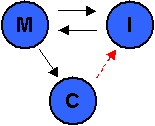# Slide Interpret

The Slide2 program consists of 3 program modules:

• MODEL
• COMPUTE
• INTERPRET

The Slide2 INTERPRET program is the post-processing module used for data visualization and interpretation of the Slide2 analysis results. This includes slope stability results as well as finite element groundwater results if you have performed a groundwater seepage analysis.

MODEL, COMPUTE and INTERPRET will each run as standalone programs. They also interact with each other as illustrated in the schematic below:• COMPUTE and INTERPRET can both be started from within MODEL
• COMPUTE must be run on a file before results can be analyzed with INTERPRET (red arrow)
• MODEL can be started from INTERPRET